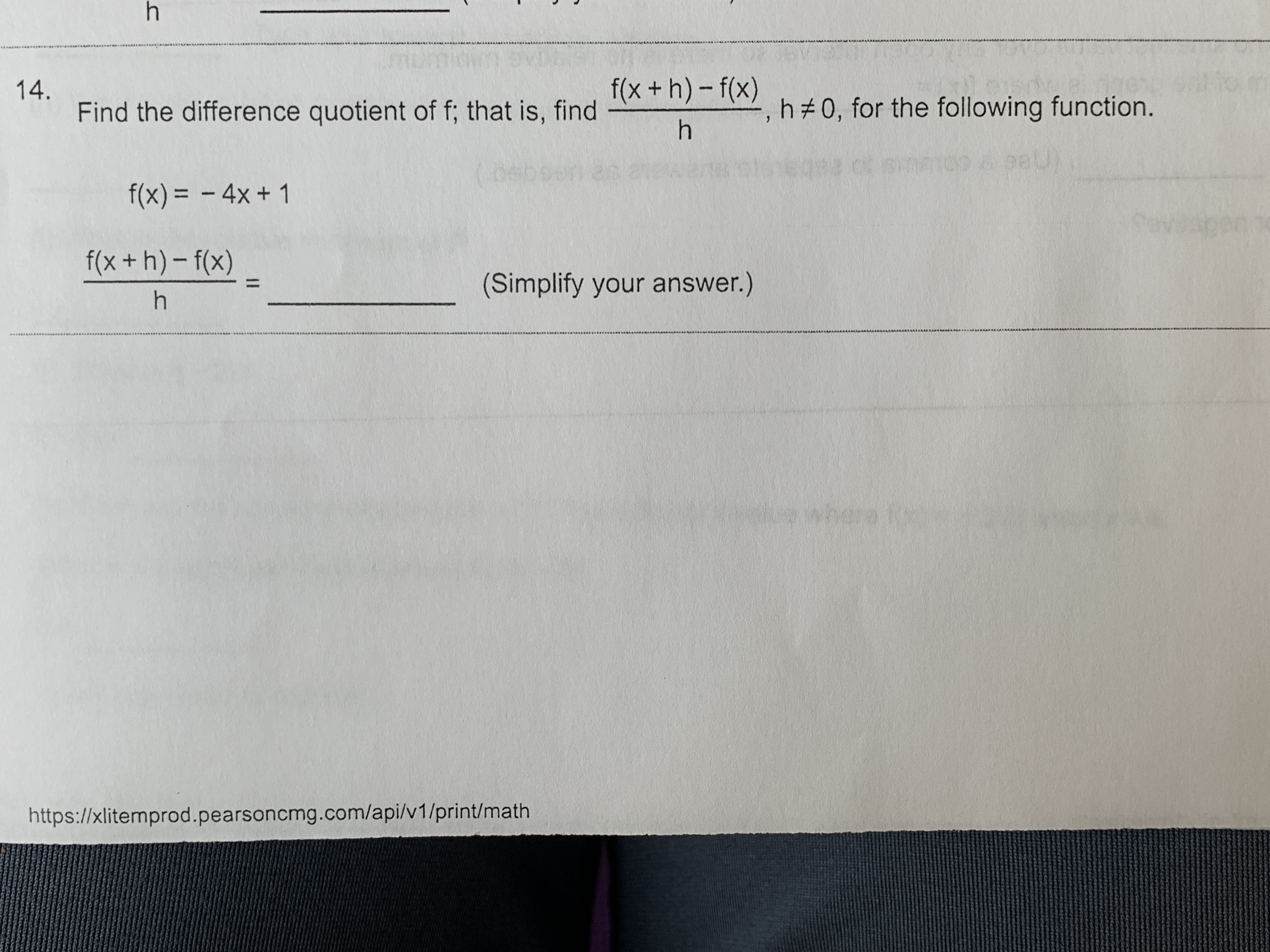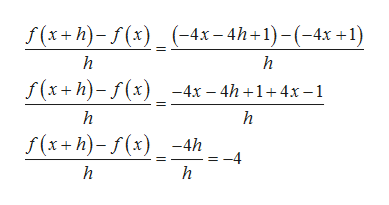# h14.Find the difference quotient of f; that is, findf(x + h) (h#0, for the following function.h(0eb40peuf(x)= 4x+ 110f(x + h)-f(x)(Simplify your answer.)hwharhttps://xlitemprod.pearsoncmg.com/api/v1/print/math

Question

Can someone help me solve this problem?help_outlineImage Transcriptioncloseh 14. Find the difference quotient of f; that is, find f(x + h) (h#0, for the following function. h (0eb 40 peu f(x)= 4x+ 1 10 f(x + h)-f(x) (Simplify your answer.) h whar https://xlitemprod.pearsoncmg.com/api/v1/print/math fullscreen
check_circle

Step 1

Firstly, we find f(x+h) by replacing x by x+h.

Step 2

Now, we find differenc...help_outlineImage TranscriptioncloseГ(*+h)-/(:) _(-4х-4h+1)-(-4х +1) h S(*+h)-/(х) _-4х - 4h +1+4х-1 h h Г(x+h)-г(х) -4h =-4 h fullscreen

### Want to see the full answer?

See Solution

#### Want to see this answer and more?

Solutions are written by subject experts who are available 24/7. Questions are typically answered within 1 hour.*

See Solution
*Response times may vary by subject and question.
Tagged in

### Other